#### How to calculate Break Even Point?

Doing a break even calculation is about ensuring that all the provided data are corresponding with the purpose of calculation. This calculation make it possible to know if any expense and profits are actually breaking even, or even if your goals are possible to accomplish. It is about making the decisions that would be beneficial to your company, and making use of strategies that are profitable for business. The break even analysis would be useful for different reasons.

There are different reasons break even analysis is valuable for the business. However, we are going to work together to do a completely break even analysis to benefit your business. I am going to use Microsoft Excel to do the break even analysis. If you are using other version of Microsoft Excel, you should basically be seeing the same thing as we work on this break even calculation.

We are going to determine which part of the business components are necessary for the break even analysis to take effect. It is not just technology that uses components, even businesses use it to know which part of the business are critical for the business to function. These components would make it possible to do the break even analysis.

The first thing you need to do is determine which areas are corresponding with the things needed to be analyzed, as you could see in the area that is marked in black. We have chosen that the unit price, unit sold, income, cost per unit, variable expenses, stable expenses, and the profit to be corresponding with making the break even calculation.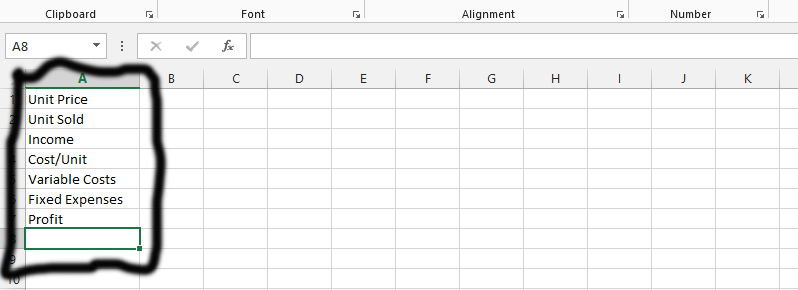We have now decided the information that we know. We already know how much the unit price is, and how many unit we have to sell, as well as the price of each unit. Finally, we do know how much our fixed expenses are, and they have already been outlaid in the picture showing below.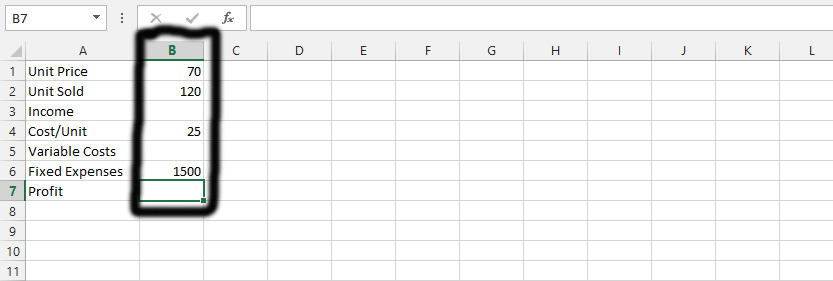Now, click on the column beside income, as it is marked and labeled as number 1, and then multiply the unit price with the quantity of sold units to know the income. This is the same thing I did with the number 2.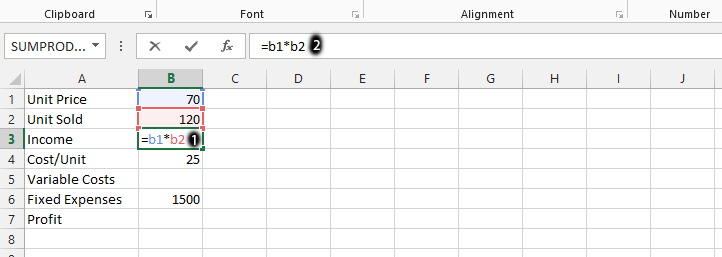You should click on the column beside the variable costs, as it is labeled as number 1, and then multiply the cost per unit with the unit sold, and then press enter.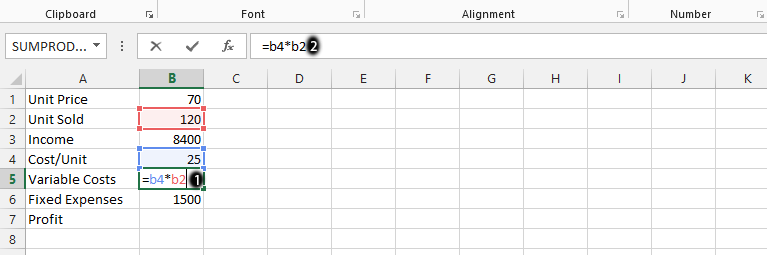Tip: You could just click on the column, type in = and use the mouse to click on the column you would like to calculate.

To find out the break even, or if we have made any profit from the business, click on the column beside profit, and type in = income – variable costs – fixed expenses, and then click enter. This is done with the one labeled as number 2.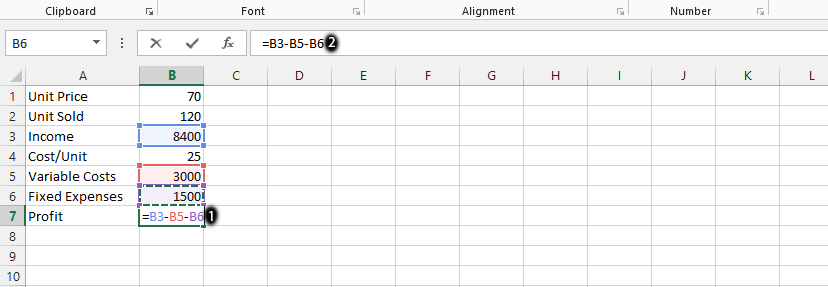Tip: You can change the profit by changing the price for each unit

## What if Analysis

This is about making some changes to effectively do the break even analysis.

Click on the Data tab, which is labeled as number 1, and then choose what if analysis, as it is labeled as number 2, and then choose goal seek. This is labeled as number 3, and marked in black as the rest of them.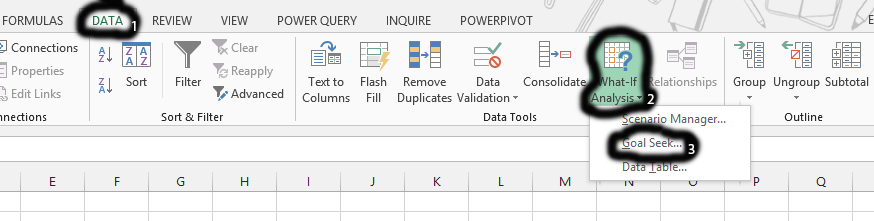Tip: Click on the column beside profit before following the step.

You should now set the one labeled as number 1, to be the one that is profit, as in this case is B7, and then you should type in 0 in the field showing in the number 2, and then type in the unit price (B1 in this case) in the one labeled as number 3, and finally click on ok, which is number 4.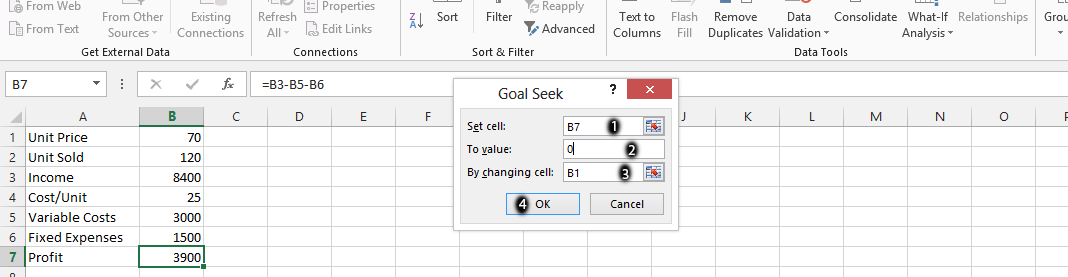You should just click okay. This means that the lowest price for you to sell your product is 37.5, anything less would make loss inevitable.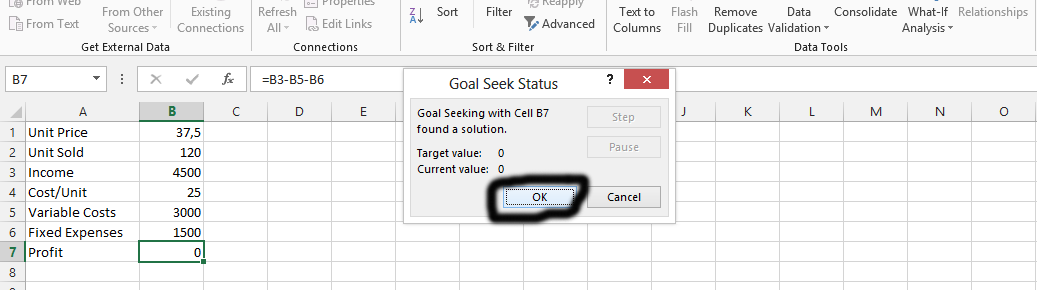This is how to calculate Break Even Point in Excel.

## How to calculate Break Even Point from chart

Calculating the Break Even Point (BEP) in Excel from a chart involves identifying the point on the chart where the total revenue line intersects with the total cost line. This point represents the level of output where the total revenue earned equals the total cost incurred, and hence, the company starts to earn a profit.

Here are the steps to calculate the Break Even Point in Excel from a chart:

1. Enter your data: Enter your data for revenue, fixed costs, and variable costs into an Excel spreadsheet.
2. Create a chart: Highlight your data and create a chart by going to the “Insert” tab and selecting the chart type that you prefer.
3. Add total revenue line: In the chart, add a new data series for the total revenue. To do this, right-click on the chart and select “Select Data”. Then click on “Add” and enter a name for the series (e.g., “Total Revenue”). For the values, enter a formula that multiplies the units of production by the price per unit.
4. Add total cost line: In the chart, add another data series for the total cost. To do this, follow the same steps as in the previous step, but this time use a formula that adds the fixed costs to the variable costs multiplied by the units of production.
5. Find the Break Even Point: The Break Even Point is the intersection point of the total revenue and total cost lines. To find this point, click on the “Layout” tab, select “Trendline”, and choose “Linear Trendline” for both the total revenue and total cost lines. Then, right-click on the chart, select “Format Trendline”, and check the box for “Display Equation on chart”. The equation for each trendline will appear on the chart. Set the two equations equal to each other, and solve for the units of production at the point of intersection. This is your Break Even Point.